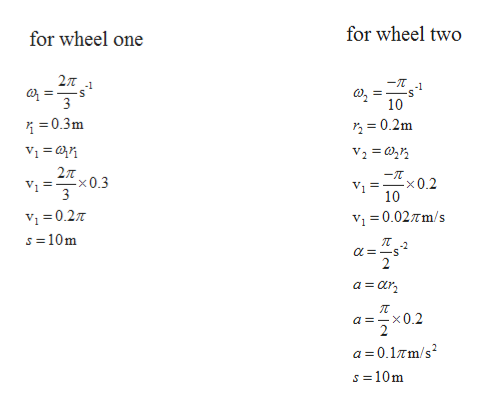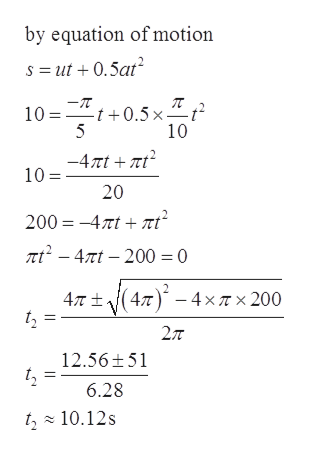# There are two wheels. Wheel one has a constant of Ω=2π/3 s^-1 and a radius of 0.3m. Wheel two has a constant a=π/2 s^-2, an initial Ω=-π/10 a d a radius of 0.2m. If these two wheels were to start at x=0, which one would travel 10m first?

Question
51 views

There are two wheels. Wheel one has a constant of Ω=2π/3 s^-1 and a radius of 0.3m. Wheel two has a constant a=π/2 s^-2, an initial Ω=-π/10 a d a radius of 0.2m. If these two wheels were to start at x=0, which one would travel 10m first?

check_circle

Step 1

Given:help_outlineImage Transcriptionclosefor wheel two for wheel one 2т л -T 1 = 3 10 0.3m 2=0.2m 27T -x 0.3 3 -T x0.2 10 Vi0.2 s =10m i 0.02m/s -2 2 a ar a =x0.2 a 0.1Tm/s s 10m fullscreen
Step 2

Time taken by first wheel

Step 3

Time taken by sec...help_outlineImage Transcriptioncloseby equation of motion s ut0.5at 10 t0.5x ^t 5 10 -4πί+ π? 10= 20 200 47tt nt2-4t-200=0 4T t(4 4xT x 200 27T 12.56 51 6.28 t, 10.12s fullscreen

### Want to see the full answer?

See Solution

#### Want to see this answer and more?

Solutions are written by subject experts who are available 24/7. Questions are typically answered within 1 hour.*

See Solution
*Response times may vary by subject and question.
Tagged in

### Physics